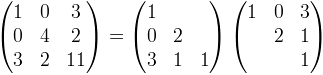﻿CholeskyDecomposition Class# CholeskyDecomposition Class

Represents the Cholesky Decomposition of a symmetric, positive definite matrix.Inheritance Hierarchy
SystemObject
Meta.Numerics.MatricesCholeskyDecomposition

Namespace:  Meta.Numerics.Matrices
Assembly:  Meta.Numerics (in Meta.Numerics.dll) Version: 4.1.4Syntax
`public sealed class CholeskyDecomposition`

The CholeskyDecomposition type exposes the following members.Properties
NameDescriptionDimension
Gets the dimension of the system.
TopMethods
NameDescriptionDeterminant
Computes the determinant of the original matrix.Equals
Determines whether the specified object is equal to the current object.
(Inherited from Object.)GetHashCode
Serves as the default hash function.
(Inherited from Object.)GetType
Gets the Type of the current instance.
(Inherited from Object.)Inverse
Computes the inverse of the original matrix.Solve
Computes the solution vector that, when multiplied by the original matrix, produces the given left-hand side vector.SquareRootMatrix
Returns the Cholesky square root matrix.ToString
Returns a string that represents the current object.
(Inherited from Object.)
TopRemarks

A Cholesky decomposition represents a matrix as the product of a lower-left triangular matrix and its transpose. For example:The Choleksy decomposition of a symmetric, positive definite matrix can be obtained using the CholeskyDecomposition method of the SymmetricMatrix class.Examples

Here is an example that uses a Cholesky decomposition to solve a linear algebra problem.

C#
```// Solve Ax = b via Cholesky decomposition
CholeskyDecomposition CD = A.CholsekyDecomposition();
ColumnVector b = new ColumnVector(1.0, 2.0, 3.0);
ColumnVector x CD.Solve(b);```See Also